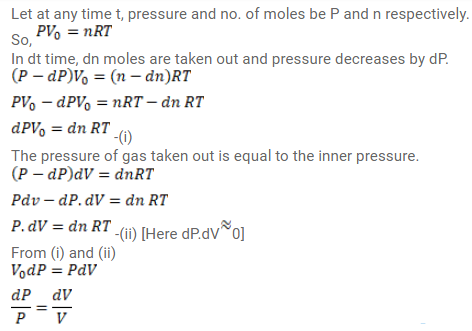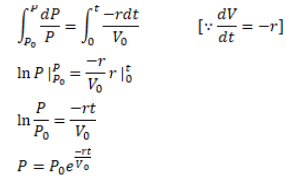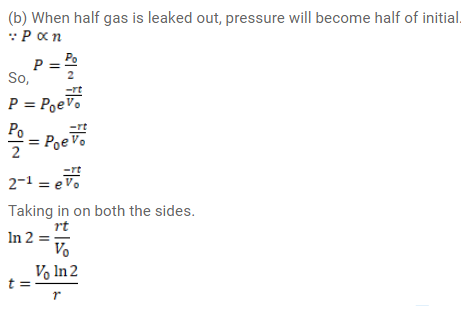# Use R=8.3 J/mol-K wherever required. A vessel of volume Vo contains an idealQuestion:

Use $\mathrm{R}=8.3 \mathrm{~J} / \mathrm{mol}-\mathrm{K}$ wherever required.

A vessel of volume $V_{0}$ contains an ideal gas at pressure $p_{0}$ and temperature $T$. Gas is continuously pumped out of this vessel at a constant-rate $\mathrm{dv} / \mathrm{dt}=\mathrm{r}$ keeping the temperature constant. The pressure of the gas being taken out equals the pressure inside the vessel. Find (a) the pressure of the gas as a function of gas, (b) the time taken before half the original gas is pumped out.

Solution: